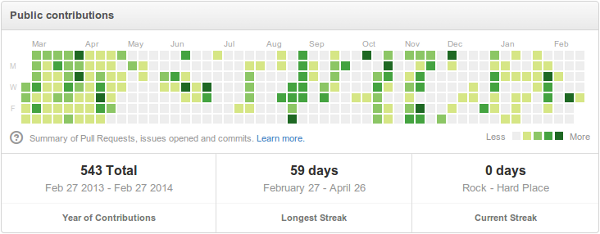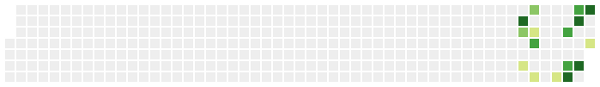# A github-like Activity Chart

One of my favorite features from github is the activity chart that appears in the user profile. For example, this one from user jmcnamara:However, I don't really use github except for one of my older projects. In part because I prefer Mercurial over git. So I decided to write a small tool to generate these charts for my own local repositories.

There are two interesting parts. First, reading the change list data from Mercurial:

``````def extract_cl_counts(repository_path, cl_counts):
"""Reads the repository changelog and extracts CL counts per day."""
repository = hg.repository(ui.ui(), repository_path)
changelog = repository.changelog
for cl_index in changelog:
cl_id = changelog.lookup(cl_index)

# The timestamp seems to be the 3rd field in the CL.
# It's given in a tuple. The UNIX timestap is the first field.
timestamp = cl
cl_date = date.fromtimestamp(timestamp)

if cl_date in cl_counts:
cl_counts[cl_date] = cl_counts[cl_date] + 1
else:
cl_counts[cl_date] = 1
``````

And then building the chart itself, which seems surprisingly easy using SVG:

``````for day_index in range(first_day_to_show, last_day_to_show):
# Boxes are stacked first by column and then by row.
x = MARGIN + (day_index // 7) * DISTANCE_BETWEEN_BOXES
y = MARGIN + (day_index % 7) * DISTANCE_BETWEEN_BOXES

# Compute the real date from the day index.
day = start_date + timedelta(days=(day_index - first_day_to_show))
if day in cl_counts:
color = get_box_color(cl_counts[day])
else:
color = get_box_color(0)